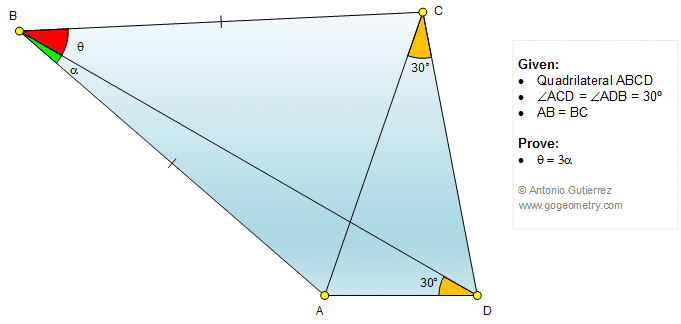# Geometry Problem 1356: Quadrilateral, Triangle, Angle, 30 Degrees, Congruence.

### Proposition

The figure below shows a quadrilateral ABCD, AB = BC and angle ACD = angle ADB = 30 degrees. Prove that angle CBD = 3(angle ABD).